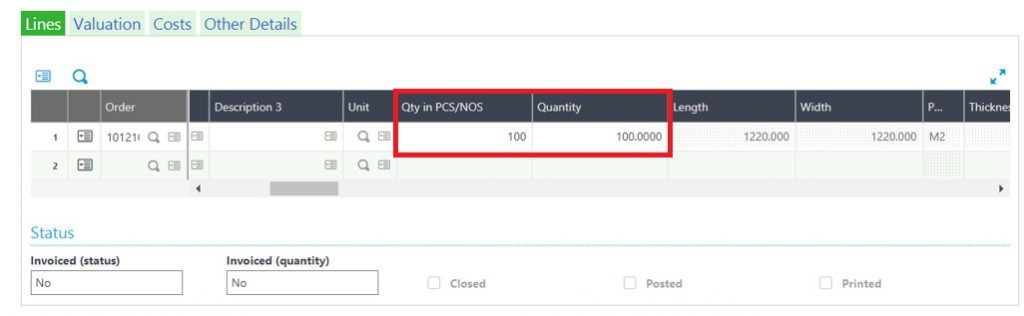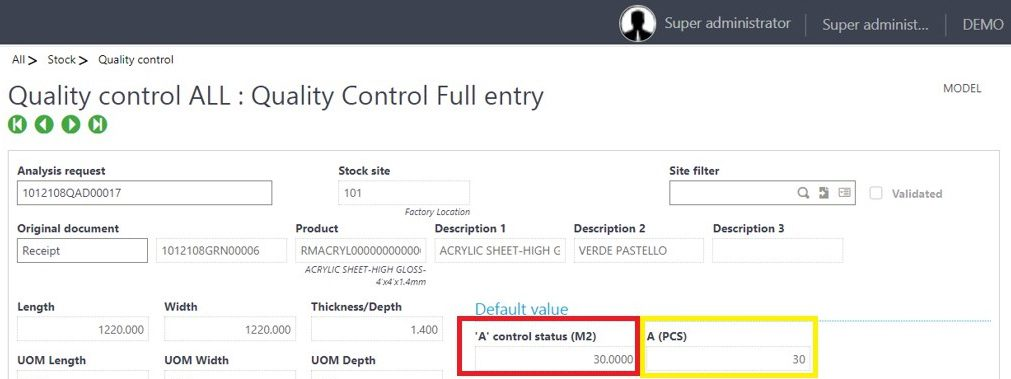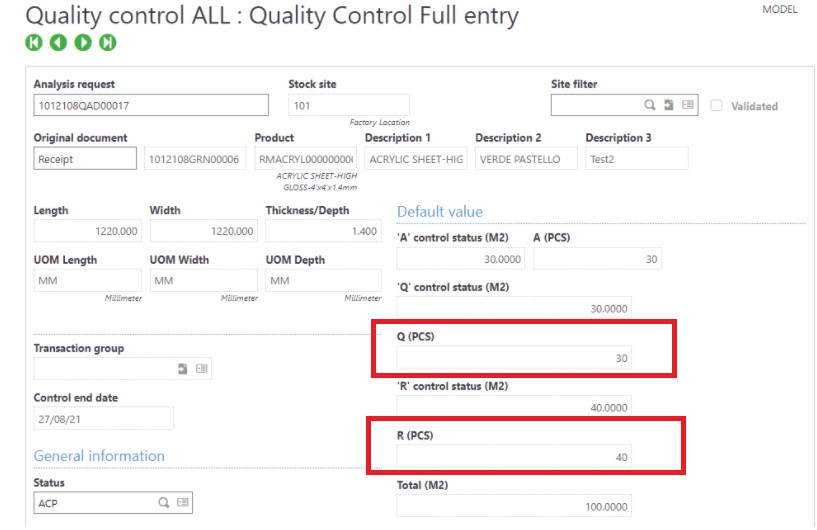# How to Check Quantity in PCS on QC Screen

By | November 30, 2021

As we all know in Sage X3, whenever Purchase Receipt/ GRN is created with status “Q” then Quality Control entry of that Purchase Receipt/ GRN will be created automatically. So that user can enter the quantity which is Accepted, rejected or still remains in Quality.

Recently we had a requirement from one of our client to check how many PCS of quantity are rejected, accepted and in quality control. As per standard functionality, in Purchase Receipt screen, there is one quantity field in detail line but for this client we have added one more quantity field i.e. Quantity in PCS. So according to this requirement, we have added three fields on the quality control screen beside Accepted, Rejected and Quality Control status quantity which will show the Quantity in PCS for accepted,rejected and quality control status

We will see the functionality in detail:

Navigate to : Purchasing -> Receipts -> Receipts Screen

New Stuff: Customization on Load WIP inquiry[Calculation of Qty in PCS]

Initially we created a Purchase Receipt as shown in the above screenshot, in which quantity in the detail line is 100 and Quntity in PCS/NOS is 100. To calculate the quanity(X) we will use formula: X value = [Qty in PCS/NOS] / [Quantity]. which means Qty in PCS/NOS will be divided by the Quantity.
If we considered the above example then 100/100 =1 i.e. X=1. So we will get X value ‘1’.

After that navigate to : Stock -> Quality Control -> Quality Control Screen[Accepted Qty in PCS]

As shown in the above Screenshot, we can see the standard field for Accepted control status which displays the overall Accepted Quantity and beside that there is a newly added field ‘A’(PCS) which will display the Accepted Quantity in PCS

Now we will check how to calculate the quantity in PCS:

If we divide the quantity of the standard ‘A’ control status field by the above particular value “X” which we have calculated using the formula – [Quantity in PCS/Quantity] then we will get the number of stocks in PCS for status “A” i.e. A(PCS) = [Quantity in ‘A’ control status / X ]. So in our example, A(PCS) = [30/1]. According to this logic A(PCS) is 30 and we can see the field marked in yellow in the above screenshot, ’30’ is getting displayed in A(PCS) field.[Quality and Rejected Qty in PCS]

Similarly, we will apply the same logic for the Quality of PCS ‘Q’(PCS) and Rejected quantity of PCS ‘R’(PCS) fields, Refer above screenshot.

In this way we can check the Quantity in PCS for Accepted, Rejected and Quality control status fields on the basis of control status fields (M2).